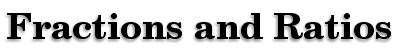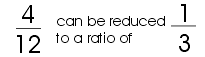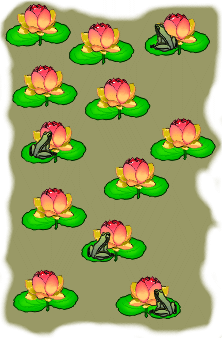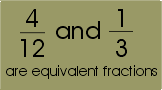Ratios are used to show the relationship between two numbers.Ratios can be written in the form of a fraction. Fractions can then be thought of as ratios. Using the illustration, we can develop a ratio of frogs to pond flowers. There are 4 frogs and 12 flowers.Equivalent fractions are fractions of equal value but represented as different ratios.

equivalent fractions

Fractions